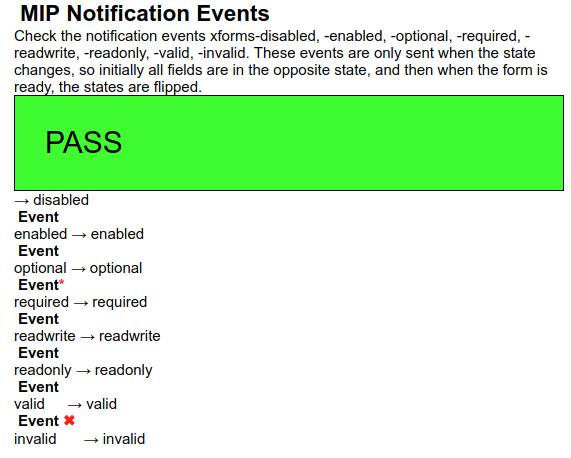## Events

In the previous example, we saw some events that happen during processing: the `xforms-ready` event that is dispatched when the system has finished initalising, and the `xforms-valid` and `-invalid` events that are dispatched when a value bound to a control changes.

In fact, when such a value changes several states are announced via events: whether the control is enabled or not, whether the value is optional or required, whether the value is readonly or not, as well as the two we have already seen. The test to check that these events are sent correctly starts by assembling test values that are all zero:

```<test pass="" res="" req="disabled">0</test>
<test pass="" res="" req="enabled">0</test>
<test pass="" res="" req="optional">0</test>
<test pass="" res="" req="required">0</test>
<test pass="" res="" req="valid">0</test>
<test pass="" res="" req="invalid">0</test>```

and binding to the values properties, so that each positive property (such as valid) is the case when the value is 1, and the opposite property (such as invalid) is the case when the value is 0:

```<bind ref="test/@req[.='enabled']"   relevant="..=1"/>
<bind ref="test/@req[.='disabled']"  relevant="..=0"/>
<bind ref="test/@req[.='valid']"     constraint="..=1"/>
<bind ref="test/@req[.='invalid']"   constraint="..=0"/>
<bind ref="test/@req[.='required']"  required="..=1"/>
<bind ref="test/@req[.='optional']"  required="..=0"/>

When initialisation is finished, all the test values are flipped to 1:

```<action ev:event="xforms-ready">
<setvalue iterate="test" ref=".">1</setvalue>
</action>```

which causes all the properties to flip. The resultant events are then caught in the output section:

```<repeat ref="test">
<output ref="@req"><label>Event</label>
<setvalue ref="../@res" ev:event="xforms-disabled" value="concat(., 'disabled')"/>
<setvalue ref="../@res" ev:event="xforms-enabled" value="concat(., 'enabled')"/>
<setvalue ref="../@res" ev:event="xforms-optional" value="concat(., 'optional')"/>
<setvalue ref="../@res" ev:event="xforms-required" value="concat(., 'required')"/>